|
|January 31, 2023

# What are S-Parameters?

The wave equation in one dimension (Telegrapher’s Equation) allows for the propagation of RF waves in both directions simultaneously within a transmission line. This makes intuitive sense if you were to imagine two signal generators launching signals, one on each end of the transmission line. The waves from each end propagate toward each other, overlap, interfere, and perhaps terminate in the source impedance of the generator on the other side.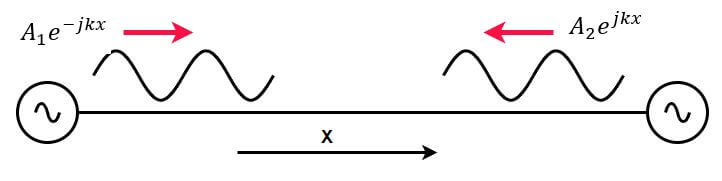Figure 1 – Two Waves on a Transmission Line

Because it is difficult to annotate two signals on a single line that are going in different directions, they are depicted using a network diagram like the one in Figure 2.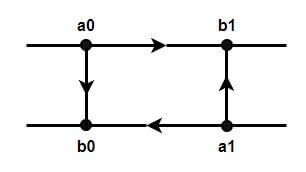Figure 2 – Network Diagram

The arrow from a0 to b1 represents a voltage wave moving from left to right, and the arrow from a1 to b0 represents a voltage wave moving from right to left. Although there are four nodes shown here, there are only two connections, and these two waves are traveling on the same line. We separate them in the network diagram for clarity and convenience. There are two interfaces shown also shown in this diagram – a0:b0 and a1:b1. Reflections may occur at these interfaces, and they are represented by the two vertical arrows.

A part of the voltage wave entering from the left of a0 may reflect at the a0-b0 interface and add to the existing wave from a1. Similarly, a reverse voltage wave entering the diagram from the right of a1 may reflect at the a1:b1 interface and add to the forward wave from a0. Waves at the top of the diagram always flow from left to right, while waves at the bottom of the diagram always flow from right to left.

These wave reflections are analogous to what occurs in optical systems where a light wave incident on a glass surface results in a reflected wave and a transmitted wave which passes into the glass.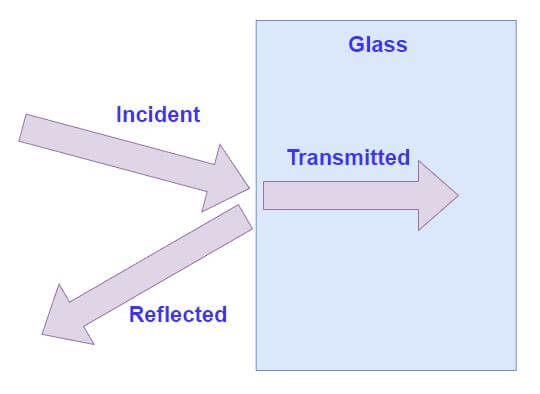Figure 3 – Optical Reflection

The reflection is proportional to the ratio of the dielectric constant of glass to that of air. If the incident power is normalized to a value of 1, then the reflected wave would be 0.2 and the transmitted wave would be 0.8.

S-parameters are normalized in this fashion as well, and the reflection is related to the impedance encountered by the wave vs the source impedance of the generator. S-parameters are complex numbers with magnitude and phase, also called reflection coefficients. The reflection, Γ from an impedance Z, fed by a generator with source impedance Zo is: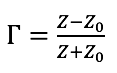This reflection always has a magnitude less than or equal to 1 and may have any phase. If Z is 0 – a short – then Γ = -1, if Z is infinite – an open – then Γ = +1.

A Vector Network Analyzer (VNA) is capable of separating and measuring the voltage waves moving in each direction on a transmission line and can determine their magnitudes and phases. An example is shown in Figure 4, where a 2-port VNA produces an outgoing incident signal on port 1, which travels to a DUT. Some of the incident signal may be reflected, and some may proceed through the DUT to arrive at port 2.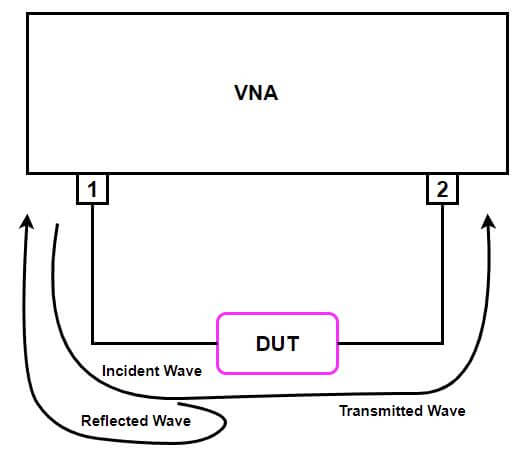Figure 4 – VNA Measurement

Based on the port voltages and currents, we can write the equations for the normalized incident and arbitrary reflected voltage waves from Figure 5 like this: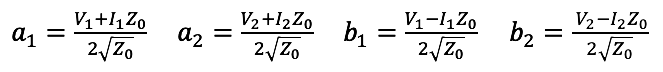Where the “a” variables are independent and “b” are dependent.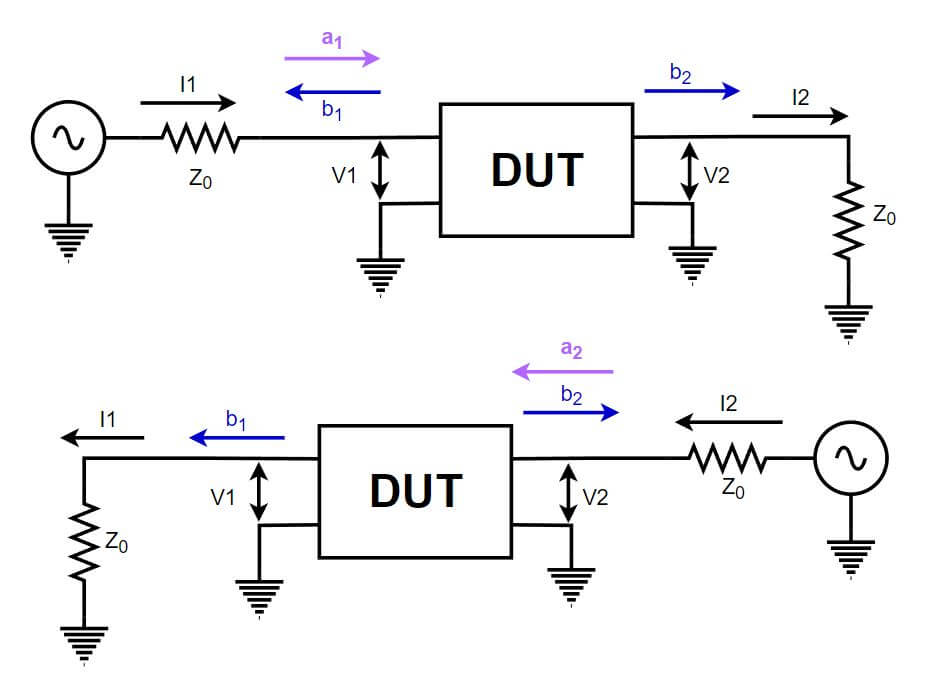Figure 5 – Port Voltages and Currents

The linear equations describing the network with S-parameter coefficients are then: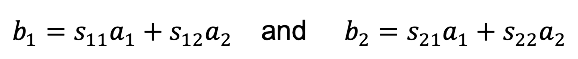And the S-parameters are defined thusly: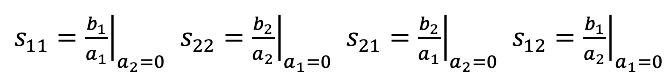Since power is proportional to the square of the voltage: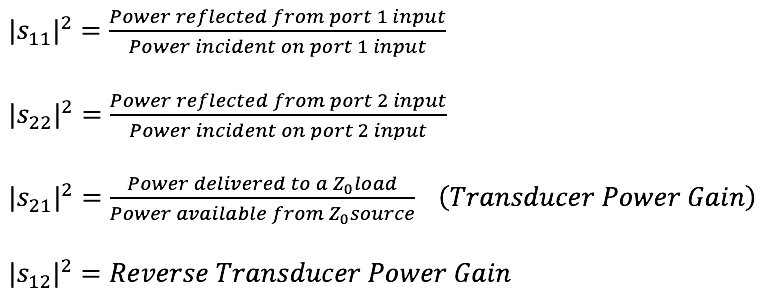S-parameters displayed in log format on a vector network analyzer portray them as 20*Log(S), which is equivalent to these power ratios.

### Why are S-Parameters Useful?

Using a Vector Network Analyzer (VNA), S-parameters may be directly measured without the need for elaborate fixturing. For instance, a 50Ω connectorized amplifier might be connected to the VNA, and the directional bridges will separate the incident and reflected waves. The return loss (S11) and transducer power gain (S21) would then be displayed on the screen.

The measurement of all four S-parameters over a frequency represents a full characterization of any linear device. S-parameter data saved as a touchstone file may be imported into most linear simulators to demonstrate the device performance within a larger system.

### Amplifiers

S-Parameters may also be used to evaluate the stability of an amplifier – see reference . We know that the input reflection coefficient, Γin, of a linear system characterized by S-parameters and loaded with output reflection coefficient ΓL is given by: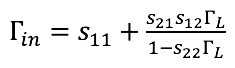The load, ΓL, has an effect on the input reflection coefficient as long as neither S21 nor S12 are zero. An amplifier will have S21 > 0 (gain) and non-zero S12 (reverse isolation). If there exists a load,  (passive load), which causes  (reflection greater than the incident wave), then the amplifier will likely oscillate with that load impedance applied. Rollet’s stability factor, K, indicates whether an amplifier is potentially unstable for some input source impedance or output load.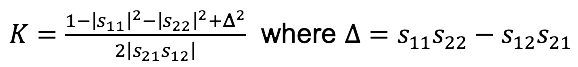An amplifier with stability factor K>1 is said to be unconditionally stable. A conditionally stable amplifier with K<1 may still be used, as long as the input and output impedances are controlled and not allowed to venture into a region where the amplifier becomes unstable.

Gain (S21), return loss (S11), 1dB compression, AM-AM conversion, and AM-PM conversion are all characteristics of an amplifier that may be evaluated with S-parameters.

### Filters

S-parameters may be used to characterize an RF filter response. A filter will have an insertion loss in the passband and one or more stopbands with required rejection. S21 is the S-parameter evaluated for these, and S11 is the reflection from the filter or return loss. See reference .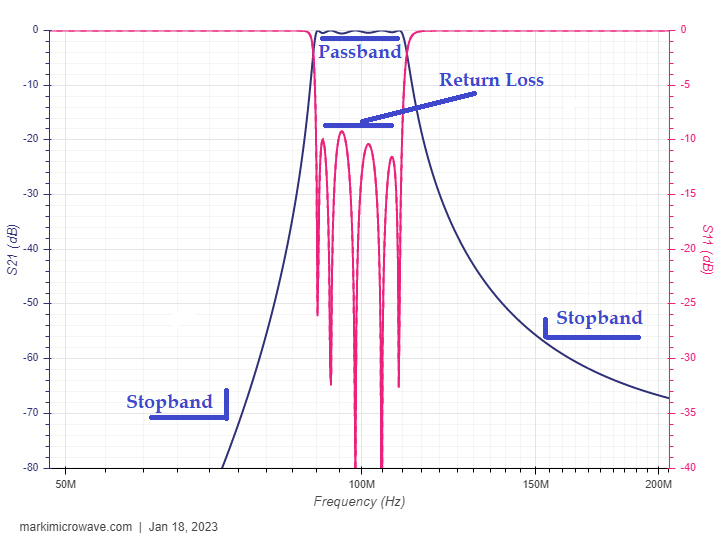Figure 6 – 100 MHz Bandpass Filter

### Transmission Lines and Antennas

Coaxial cables and balanced transmission lines connect RF devices to each other and to antennas. Measuring S21 (insertion loss) of these lines may be important to determine how much RF energy is being transferred to the load at the end of the line. Poor return loss could indicate a damaged cable or failing connector. The measurement of the return loss looking into a cable that feeds an antenna is an effective way to evaluate the health of both. Physical damage to the antenna or ingress of moisture into the feed-line cable increases reflection and degrades the return loss. See references  and .

### Material Properties and Moisture Content

mmWave S-Parameter measurements of materials may be used to derive the permittivity of unknown materials. This information is critical for the development of radomes for aerospace and automotive applications. See reference .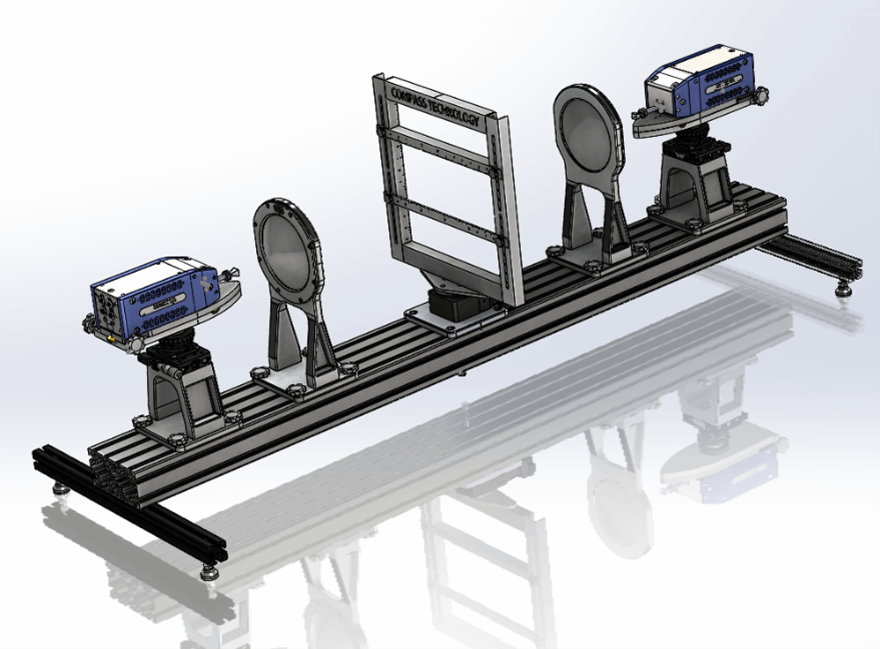Figure 7 – Material Measurement from Compass Technology Group

Because the relative permittivity of water is very high – 80 – it raises the overall relative permittivity of any material containing it. Dry soil might have a relative permittivity between 3 and 5, but added moisture drives this number up very quickly, so it is possible to estimate moisture content. Soil moisture estimation is extremely important in the agricultural industry. See reference .

## Conclusion

S-parameters are very useful for a great number of RF measurements. With the advent of the modern VNA, there is simply no reason to use the older characterization parameters such as Z, Y, or h. These older parameters require special fixturing and are not suitable for high-frequency measurement. S-parameters can characterize devices up to hundreds of GHz.

For more information on measurement techniques and VNA equipment to perform those measurements, please see our website at Copper Mountain Technologies, www.coppermountaintech.com.